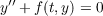#### Vol. 24, No. 1, 1968

 Download this articleFor screen For printingRecent Issues Vol. 325: 1  2 Vol. 324: 1  2 Vol. 323: 1  2 Vol. 322: 1  2 Vol. 321: 1  2 Vol. 320: 1  2 Vol. 319: 1  2 Vol. 318: 1  2Online Archive Volume: Issue:The Journal Subscriptions Editorial Board Officers Contacts Submission Guidelines Submission Form Policies for Authors ISSN: 1945-5844 (e-only) ISSN: 0030-8730 (print) Special Issues Author Index To Appear Other MSP Journals
Oscillation of solutions to second-order nonlinear differential equations

### Jack W. Macki and James Sai-Wing Wong

Vol. 24 (1968), No. 1, 111–117
##### Abstract

A solution y(t) of(1)

is said to be oscillatory if for every T > 0 there exists t0 > T such that y(t0) = 0. Let be the class of solutions of (1) which are indefinitely continuable to the right, i.e. y ∈ℱ implies y(t) exists as a solution to (1) on some interval of the form [Ty,). Equation (1) is said to be oscillatory if each solution from is oscillatory. If no solution in is oscillatory, equation (1) is said to be nonoscillatory.

THEOREM 1. Let f(t,x) be continuous and satisfy b(t)Ψ(x) f(t,x) a(t)Φ(αj) for 0 t < , −∞ < x < , where

1. a(t) 0,b(t) 0 are both locally integrable,
2. Φ(x),Ψ(x) are nondecreasing and satisfy xΦ(x) > 0, xΨ(x) > 0 for x0 and, for some α 0, α[Φ(u)]1 du < , α−∞[Ψ(u)]1 du < . Then equation (1) is oscillatory if and only if ta(t)dt = tb(t)dt = .

Conditions on f(t,x) are also given (Theorem 2) which are sufficient for equation (1) to be nonoscillatory.

Primary: 34.42
##### Milestones